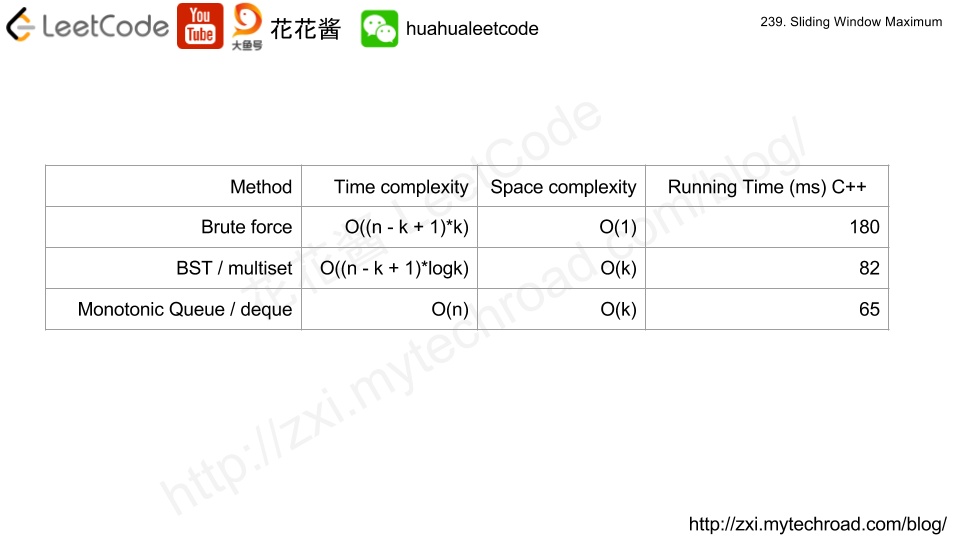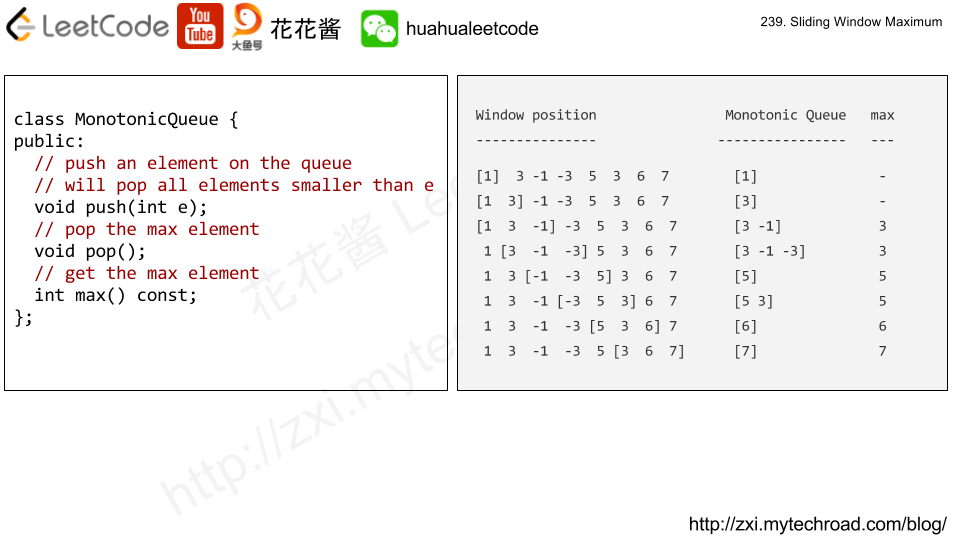# Posts tagged as “heap”

You are driving a vehicle that has capacity empty seats initially available for passengers.  The vehicle only drives east (ie. it cannot turn around and drive west.)

Given a list of tripstrip[i] = [num_passengers, start_location, end_location] contains information about the i-th trip: the number of passengers that must be picked up, and the locations to pick them up and drop them off.  The locations are given as the number of kilometers due east from your vehicle’s initial location.

Return true if and only if it is possible to pick up and drop off all passengers for all the given trips.

Example 1:

Input: trips = [[2,1,5],[3,3,7]], capacity = 4
Output: false


Example 2:

Input: trips = [[2,1,5],[3,3,7]], capacity = 5
Output: true


Example 3:

Input: trips = [[2,1,5],[3,5,7]], capacity = 3
Output: true


Example 4:

Input: trips = [[3,2,7],[3,7,9],[8,3,9]], capacity = 11
Output: true

## Solution1: Min heap

Sort events by location

Time complexity: O(nlogn)
Space complexity: O(n)

## Solution 2: Preprocessing

Time complexity: O(n)
Space complexity: O(1000)

# Problem

Merge k sorted linked lists and return it as one sorted list. Analyze and describe its complexity.

Example:

Input:
[
1->4->5,
1->3->4,
2->6
]
Output: 1->1->2->3->4->4->5->6

# Solution 1: Brute Force

Time complexity: O(nk)

Space complexity: O(1)

# Solution 2: Heap / Priority Queue

Time complexity: O(nlogk)

Space complexity: O(k)

# Problem

Given an integer array, find three numbers whose product is maximum and output the maximum product.

Example 1:

Input: [1,2,3]
Output: 6


Example 2:

Input: [1,2,3,4]
Output: 24


Note:

1. The length of the given array will be in range [3,104] and all elements are in the range [-1000, 1000].
2. Multiplication of any three numbers in the input won’t exceed the range of 32-bit signed integer.

# Idea:

Find the top 3 numbers t1, t2, t3, and bottom 2 numbers, b1, b2.

If all numbers are positives,  answer must be t1 * t2 * t3.

Since the number can go negative, the answer must be either t1*t2*t3 or b1 * b2 * t1, if b1 and b2 are both negatives.

ex. nums: [5, 1, -6, 3, -1]

t1, t2, t3: 5, 3, 1

b1, b2: -6, -1

t1 * t2 * t3 = 15

t1 * b1 * b2 = 30

# Solution 1: Manual Tracking

Time complexity: O(n)

Space complexity: O(1)

# Solution 2: Sorting

Time complexity: O(nlogn)

Space complexity: O(1)

# Solution 3: Two Heaps (Priority Queues)

Time complexity: O(nlog3)

Space complexity: O(2 + 3)

# Problem

Design a class to find the kth largest element in a stream. Note that it is the kth largest element in the sorted order, not the kth distinct element.

Your KthLargest class will have a constructor which accepts an integer k and an integer array nums, which contains initial elements from the stream. For each call to the method KthLargest.add, return the element representing the kth largest element in the stream.

Example:

int k = 3;
int[] arr = [4,5,8,2];
KthLargest kthLargest = new KthLargest(3, arr);


Note:
You may assume that nums‘ length ≥ k-1 and k ≥ 1.

# Solution: BST / Min Heap

Time complexity: O(nlogk)

Space complexity: O(k)

C++ / BST

C++ / Min Heap

Problem:

https://leetcode.com/problems/sliding-window-maximum/

Given an array nums, there is a sliding window of size k which is moving from the very left of the array to the very right. You can only see the k numbers in the window. Each time the sliding window moves right by one position.

For example,
Given nums = [1,3,-1,-3,5,3,6,7], and k = 3.

Therefore, return the max sliding window as [3,3,5,5,6,7].

Note:
You may assume k is always valid, ie: 1 ≤ k ≤ input array’s size for non-empty array.

Could you solve it in linear time?

Idea:# Solution 1: Brute Force

Time complexity: O((n – k + 1) * k)

Space complexity: O(1)

# Solution 2: BST

Time complexity: O((n – k + 1) * logk)

Space complexity: O(k)

# Solution 3: Monotonic Queue

Time complexity: O(n)

Space complexity: O(k)

## Python3 V2

Mission News Theme by Compete Themes.